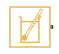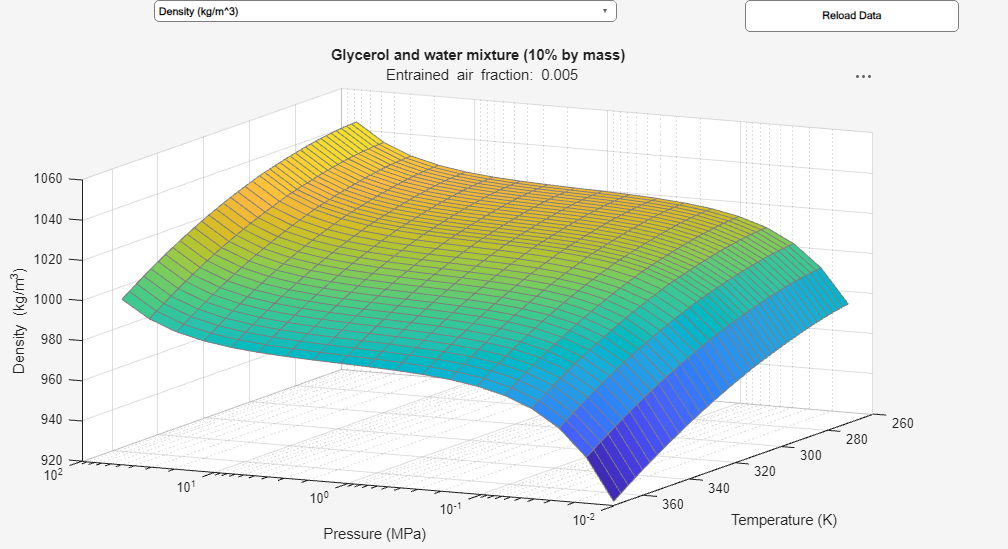# Thermal Liquid Properties (TL)

Preset fluid properties for the simulation of a thermal liquid network

•Libraries:
Simscape / Fluids / Thermal Liquid / Utilities

## Description

The Thermal Liquid Properties (TL) block set predefined fluid properties to a thermal liquid network. The available fluids include pure water, aqueous mixtures, diesel, aviation fuel Jet A, and SAE 5W-30. You can use this block as a preset alternative to the Thermal Liquid Settings (TL) block. If your network does not have a connected liquid properties block, or the liquid defaults will apply. See Specify Fluid Properties for more details

The preset fluid properties are defined in tabular form as functions of temperature and pressure. During simulation, the network properties are set by linear interpolation between data points. Tabular data for aqueous mixtures is provided for concentration by mass or volume.

All the fluid properties commonly specified in the Thermal Liquid Settings (TL) block are defined in the block. These properties include density, the bulk modulus and thermal expansion coefficient, the specific internal energy and specific heat, as well as the kinematic viscosity and thermal conductivity. The properties are valid over a limited region of temperatures and pressures specific to the fluid selected and dependent, in the cases of mixtures, on the concentration specified. Simulation is allowed within this validity region only.

### Data Visualization

You can visualize the fluid properties defined in the block and the pressure and temperature regions of validity. To open the visualization utility, right-click the block and select Fluids > Plot Fluid Properties. The plot updates automatically upon selection of a fluid property from the drop-down list. Use the button to regenerate the plot whenever the fluid selection or fluid parameters change.

Visualization of density data for a 10% glycerol aqueous mixture### Validity Regions

The validity regions are defined in the block as matrices of zeros and ones. Each row corresponds to a tabulated temperature and each column to a tabulated pressure. A zero denotes an invalid breakpoint and a one a valid breakpoint. These validity matrices are internal to the block and cannot be modified; they can only be checked (using the data visualization utility of the block).

In most cases, the validity matrices are extracted directly from the tabulated data. The glycol and glycerol mixtures pressure boundaries are not available from the data, and are obtained explicitly from block parameters. The figure below shows an example of the validity region for water. The shaded squares indicate temperature and pressure regions outside of the validity region.`Water`

The properties of water are valid at temperatures above the triple-point value (`273.160 K`) up to the critical-point value (`647.096 K`). They are valid at pressures above the greater of the triple-point value (`611.657 Pa`) on one hand and the temperature-dependent saturation value on the other, up to the critical-point value (`22.064 MPa`). Pressures below the saturation point for a given temperature row are assigned a value of `0` in the validity matrix.

`Seawater (MIT model)`

The properties of seawater are valid at temperatures above `0°C` up to `120°C` (```273.15 K``` to `393.15 K`); they are valid at pressures above the saturation point up to a maximum value of `12 MPa`. Pressures below the saturation point for a given temperature row (and at the specified concentration level) are assigned a value of `0` in the validity matrix. Mixture concentrations can range in value from `0` to `0.12` on a mass fraction basis.

`Ethylene glycol and water mixture`

The properties of an aqueous ethylene glycol mixture are valid over a temperature domain determined from the mixture concentration; they are valid at pressures within the minimum and maximum bounds specified in the block dialog box (extended horizontally to span the width of the temperature rows).

The lower temperature bound is always the lesser of the minimum temperature extracted from the available data and the freezing point of the mixture (the mixture must be in the liquid state). The upper temperature bound is always the maximum temperature extracted from the data. Mixture concentrations can range in value from `0` to `0.6` if a mass-fraction basis is used, or from `0` to `1` if a volume fraction basis is used.

`Propylene glycol and water mixture`

The properties of an aqueous propylene glycol mixture are valid over the temperature and pressure ranges described for the case of ```Ethylene glycol and water mixture```. Mixture concentrations can range in value from `0` to `0.6` if a mass-fraction basis is used, or from `0.1` to `0.6` if a volume fraction basis is used.

`Glycerol and water mixture`

The properties of an aqueous glycerol mixture are valid over the temperature and pressure ranges as described for the case of ```Ethylene glycol and water mixture```. Mixture concentrations can range in value from `0` to `0.6` on a mass-fraction basis. The properties are all extended to `100 degC` as:

• Alpha (density thermal derivative) — linear fit

• Specific heat — linear fit

• Specific internal energy — linear fit

• Kinematic viscosity — exponential fit based on the upper half of the original temperature range

• Dynamic viscosity — exponential fit based on the upper half of the original temperature range

• Prandtl number — exponential fit based on the upper half of the original temperature range

`Aviation fuel Jet-A`

The properties of Jet A fuel are valid at temperatures above `-50.93°C` up to `372.46°C` (`222.22 K` to `645.61 K`); they are valid at pressures above the saturation point up to a maximum value of ```2.41 MPa```. Pressures below the saturation point for a given temperature row are assigned a value of `0` in the validity matrix.

`Diesel fuel`

The properties of diesel fuel are valid at temperatures above `-34.95°C` up to `417.82°C` (`238.20 K` to `690.97 K`); they are valid at pressures above the saturation point up to a maximum value of ```2.29 MPa```. Pressures below the saturation point for a given temperature row are assigned a value of `0` in the validity matrix.

`SAE 5W-30`

The properties of SAE 5W-30 fuel derive from data that cover temperature and pressure ranges for each property. The block uses measured properties between `29.85°C` and `74.85°C` (```303 K``` to ` 348 K`) and `7 MPa` and `82 MPa`. The block extrapolates data outside of this region to `-38°C` and `200°C` (```235.15 K``` and `473.15 K`) and to ```0.01 MPa``` and `100 MPa`. The way the block smooths and extrapolates the data depends on the type of data:

• Density — The block uses a quadratic fit. The block fits extrapolated regions to the bulk modulus and alpha fundamental relationships.

• Bulk modulus — The block uses fundamental relationship with density based on the density quadratic fit. The block uses linear extrapolation in the extrapolation region.

• Alpha (density thermal derivative) — The block uses the fundamental relationship with density based on density quadratic fit. The block uses linear extrapolation in the extrapolation region.

• Specific heat — The block uses linear extrapolation in the extrapolation region. There is no dependency on pressure.

• Thermal conductivity — The block uses linear extrapolation in the extrapolation region. There is no dependency on pressure.

• Kinematic viscosity — The block uses the fundamental relationship with the fits of dynamic viscosity and density.

• Dynamic viscosity — The block uses an exponential fit with temperature and a linear fit with pressure.

• Prandtl number — The block uses the fundamental relationship with the fits of specific heat, dynamic viscosity, and thermal conductivity.

### Calculated Properties

The density and thermal expansion coefficients of the aqueous mixtures of glycol and glycerol compounds are obtained from the block parameters. The fluid density, with respect to pressure and temperature, is calculated as:

`$\rho \left(T,p\right)=\rho \left(T\right)\text{exp}\left(\frac{p-{p}_{\text{R}}}{\beta }\right),$`

where:

• T is the network temperature.

• p is the network pressure.

• ρ is the fluid density.

• pR is the reference pressure associated with the fluid property tables.

• ß is the isothermal bulk modulus.

where the change in fluid density is evaluated as:

`${\left(\frac{\partial \rho \left(T,p\right)}{\partial T}\right)}_{p}={\left(\frac{\partial \rho \left(T\right)}{\partial T}\right)}_{T}\text{exp}\left(\frac{p-{p}_{\text{R}}}{\beta }\right).$`

The thermal expansion coefficient is calculated as:

`$\alpha \left(T,p\right)=-\frac{1}{\rho \left(T,p\right)}{\left(\frac{\partial \rho \left(T\right)}{\partial T}\right)}_{T}\text{exp}\left(\frac{p-{p}_{\text{R}}}{\beta }\right).$`

## Ports

### Conserving

expand all

Node identifying the thermal liquid network for which to define the necessary fluid properties. The fluid selected in this block applies to the entire network. No other Thermal Liquid Properties (TL) or Thermal Liquid Settings (TL) block may be connected to the same network.

## Parameters

expand all

Sets the fluid properties of your liquid network. The available fluids include pure water, aqueous mixtures, motor oils, and fuels.

Ratio of the mass of salt present in the saline mixture to the total mass of that mixture.

#### Dependencies

This parameter is active only when ```Seawater (MIT model)``` is selected as the working fluid.

Quantity in terms of which to specify the concentration of ethylene glycol in its aqueous mixture. This parameter is active only when either `Ethylene glycol and water mixture` or `Propylene glycol and water mixture` is selected as the working fluid.

Volume of ethylene glycol present in the aqueous mixture divided by the total volume of that mixture.

#### Dependencies

This parameter is active when ```Ethylene glycol and water mixture``` is selected as the working fluid and `Volume fraction` is selected as the concentration type.

Mass of ethylene glycol present in the aqueous mixture divided by the total mass of that mixture.

#### Dependencies

This parameter is active when ```Ethylene glycol and water mixture``` is selected as the working fluid and `Mass fraction` is selected as the concentration type.

Volume of propylene glycol present in the aqueous mixture divided by the total volume of that mixture.

#### Dependencies

This parameter is active when ```Propylene glycol and water mixture``` is selected as the working fluid and `Volume fraction` is selected as the concentration type.

Mass of propylene glycol present in the aqueous mixture divided by the total mass of that mixture.

#### Dependencies

This parameter is active when ```Propylene glycol and water mixture``` is selected as the working fluid and `Mass fraction` is selected as the concentration type.

Bulk modulus of the aqueous mixture at constant temperature. The bulk modulus measures the change in pressure required to produce a fractional change in fluid volume.

#### Dependencies

This parameter is active when either ```Ethylene glycol and water mixture```, ```Propylene glycol and water mixture```, or ```Glycerol and water mixture``` is selected as the working fluid.

Lower bound of the pressure range allowed in the thermal liquid network connected to this block.

#### Dependencies

This parameter is active when either ```Ethylene glycol and water mixture```, ```Propylene glycol and water mixture```, or ```Glycerol and water mixture``` is selected as the working fluid.

Upper bound of the pressure range allowed in the thermal liquid network connected to this block.

#### Dependencies

This parameter is active when either ```Ethylene glycol and water mixture```, ```Propylene glycol and water mixture```, or ```Glycerol and water mixture``` is selected as the working fluid.

Absolute pressure of the external environment in which the thermal liquid network is assumed to run. The default value is the standard atmospheric pressure measured at sea level on Earth.

Select what happens if the fluid pressure or temperature go beyond the valid range during simulation:

• `None` ― The block does not return an error if the properties go out of range.

• `Warning` ― The block issues a warning, but continues the simulation.

• `Error` ― The block returns an error and stops the simulation.

 Massachusetts Institute of Technology (MIT), Thermophysical properties of seawater database. http://web.mit.edu/seawater.

 K.G. Nayar, M.H. Sharqawy, L.D. Banchik, J.H. Lienhard V, Thermophysical properties of seawater: A review and new correlations that include pressure dependence, Desalination, Vol. 390, pp. 1-24, 2016.

 M.H. Sharqawy, J.H. Lienhard V, S.M. Zubair, Thermophysical properties of seawater: A review of existing correlations and data, Desalination and Water Treatment, Vol. 16, pp. 354-380.